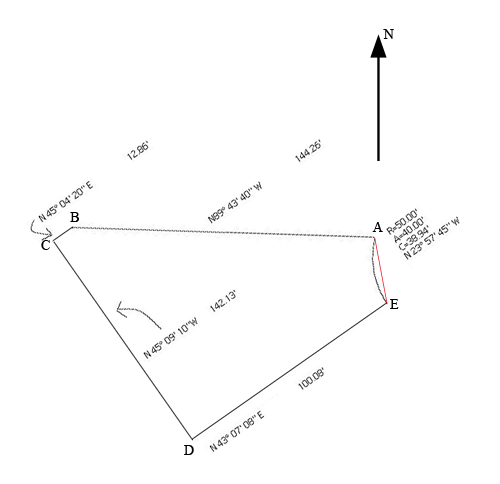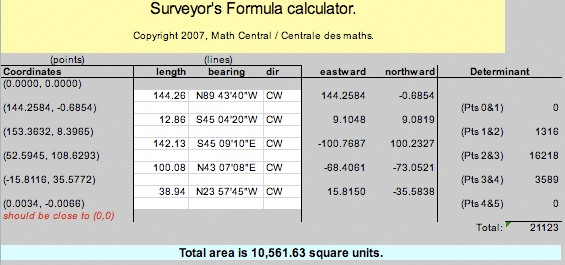SEARCH HOMEMath Central Quandaries & QueriesQuestion from Chris: Hi - I'm trying out to figure out how to calculate the size of an odd shaped lot with a curve to it. Any help would be appreciated. Thanks for any help. ChrisChris,

I rotated the diagram you sent to put north at the top, I added labels at the vertices and joined E and A with a straight line.I then used our Surveyor's Formula calculator to find the area of the property where the boundary between E and A is a straight line of length 38.94 feet.You will see that I changed some of the bearings to simulate walking around the property in a counterclockwise direction starting at A. For example when you are at B then C is south west of you. The area obtained is 10,561.63 square feet.

I then needed to remove the area of the circular bite taken out of the property by the arc EA. The radius of the circle is R = 50.0 feet and my calculations gave the area of the circular bite removed to be 103.29 square feet. The area of the lot is then 10,561.63 - 103.29 = 10,458.34 square feet. There are 43,560 square feet in an acre so that's 10,458.34/43,560 = 0.24 acres.